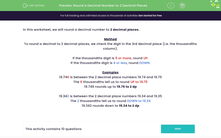# Round a Decimal Number to 2 Decimal Places

In this worksheet, students will round a decimal number to 2 decimal places.Key stage:  KS 3

Curriculum topic:   Number

Curriculum subtopic:   Round/Approximate Numbers Appropriately

Popular topics:   Decimals worksheets

Difficulty level:#### Worksheet Overview

In this activity, we will round a decimal number to 2 decimal places.

Method

To round a decimal to 2 decimal places, we check the digit in the 3rd decimal place (i.e. the thousandths column).

If the thousandths digit is 5 or more, round UP.

If the thousandths digit is 4 or less, round DOWN.

Examples

19.746 is between the 2 decimal place numbers 19.74 and 19.75

The 6 thousandths tell us to round UP to 19.75

19.746 rounds up to 19.75 to 2 dp

19.342 is between the 2 decimal place numbers 19.34 and 19.35

The 2 thousandths tell us to round DOWN to 19.34

19.342 rounds down to 19.34 to 2 dp

Are you ready to have a go at some questions now?### What is EdPlace?

We're your National Curriculum aligned online education content provider helping each child succeed in English, maths and science from year 1 to GCSE. With an EdPlace account you’ll be able to track and measure progress, helping each child achieve their best. We build confidence and attainment by personalising each child’s learning at a level that suits them.

Get started